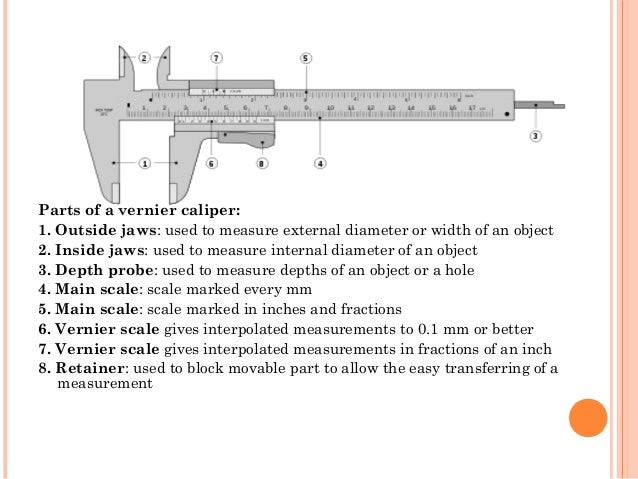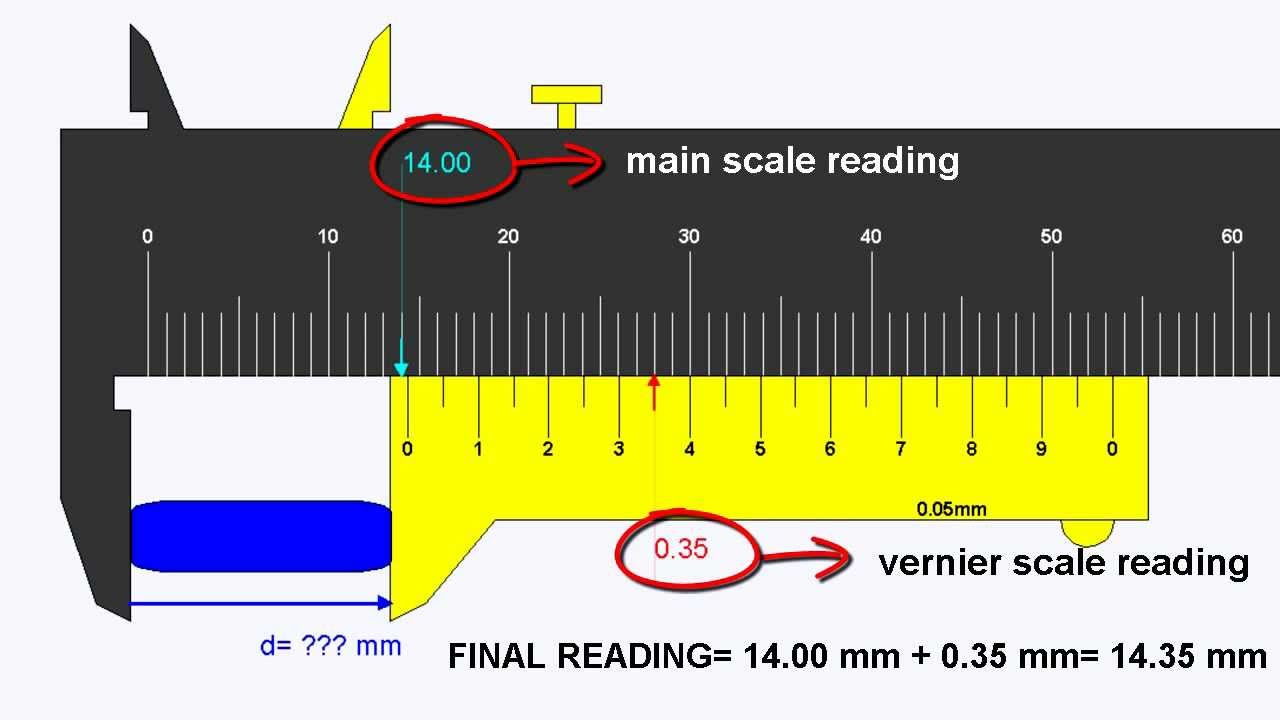## Translate

### What is Vernier Caliper? How to Use it ?VERNIER CALLIPERS:-

Vernier calipers, an instrument for making very accurate linear measurements was introduced in 1631 by Pierre Vernier of France. Vernier calipers are widely used in scientific laboratories and in manufacturing for quality control measurements.

Least count of the instrument :

It is defined as the minimum reading that can be read/taken from the measuring instrument.

or

The least count of an instrument is the smallest measurement that can be taken accurately with it.

Principle of Vernier :-

The graduations on the vernier scale are such that the length of ‘ n’ divisions on the vernier scale is equal to (n-1) divisions of the main scale. Generally, a vernier has 10 divisions and the the length of these 10 divisions is equal to the length of 10 – 1 = 9 divisions of the main scale.

That is , 10 div of vernier scale = 9 mm.
1 div of vernier scale = 9/10 mm

Parts of Vernier Caliper :-Uses of a vernier calipers :-

Vernier callipers are used to measure,

(i)The length of a rod or any object
(ii)The diameter of a sphere
(iii)The internal and external diameter of a hollow cylinder
(iv)The depth of a small beaker

How to use a Vernier calliper :-

1) The jaws are first gently closed on the object to be measured.
2) Note the main scale reading (M.S.R)
3) Note the division on vernier scale which coincides with any division of the main scale. Multiply this number of vernier division with the least count. This is the vernier scale reading(V.S.R) Hence V.S.R = Vernier scale coincidence x Least count(L.C.)
4) Add the main scale reading to the vernier scale reading. This gives the observed length.

Hence, Observed Reading = Main scale reading + Vernier scale reading.

Observed reading = M.S.R + V.S.R
OR
Observed reading = M.S.R. + (Vernier scale coincidence x L.C.)